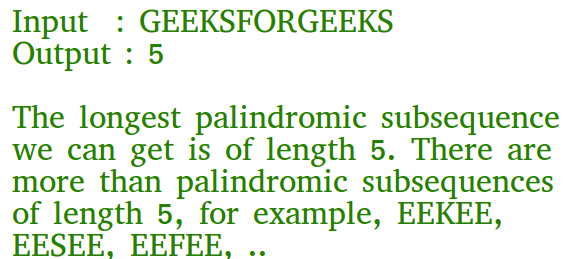# PHP Program for Longest Palindromic Subsequence | DP-12

• Last Updated : 13 Aug, 2021

Given a sequence, find the length of the longest palindromic subsequence in it.As another example, if the given sequence is “BBABCBCAB”, then the output should be 7 as “BABCBAB” is the longest palindromic subsequence in it. “BBBBB” and “BBCBB” are also palindromic subsequences of the given sequence, but not the longest ones.

1) Optimal Substructure:
Let X[0..n-1] be the input sequence of length n and L(0, n-1) be the length of the longest palindromic subsequence of X[0..n-1].
If last and first characters of X are same, then L(0, n-1) = L(1, n-2) + 2.
Else L(0, n-1) = MAX (L(1, n-1), L(0, n-2)).
Following is a general recursive solution with all cases handled.

Dynamic Programming Solution

## PHP

 ` \$y)? \$x : \$y; }`   `// Returns the length of the` `// longest palindromic ` `// subsequence in seq` `function` `lps(``\$str``)` `{` `\$n` `= ``strlen``(``\$str``);` `\$i``; ``\$j``; ``\$cl``;`   `// Create a table to store` `// results of subproblems` `\$L``[][] = ``array``(``array``()); `     `// Strings of length 1 are` `// palindrome of length 1` `for` `(``\$i` `= 0; ``\$i` `< ``\$n``; ``\$i``++)` `    ``\$L``[``\$i``][``\$i``] = 1;`   `    ``// Build the table. Note that ` `    ``// the lower diagonal values ` `    ``// of table are useless and ` `    ``// not filled in the process. ` `    ``// The values are filled in a ` `    ``// manner similar to Matrix ` `    ``// Chain Multiplication DP ` `    ``// solution (See ` `    ``// https://www.geeksforgeeks.org/matrix-chain-multiplication-dp-8/).` `    ``// cl is length of substring` `    ``for` `(``\$cl` `= 2; ``\$cl` `<= ``\$n``; ``\$cl``++)` `    ``{` `        ``for` `(``\$i` `= 0; ``\$i` `< ``\$n` `- ``\$cl` `+ 1; ``\$i``++)` `        ``{` `            ``\$j` `= ``\$i` `+ ``\$cl` `- 1;` `            ``if` `(``\$str``[``\$i``] == ``\$str``[``\$j``] && ` `                            ``\$cl` `== 2)` `            ``\$L``[``\$i``][``\$j``] = 2;` `            ``else` `if` `(``\$str``[``\$i``] == ``\$str``[``\$j``])` `            ``\$L``[``\$i``][``\$j``] = ``\$L``[``\$i` `+ 1][``\$j` `- 1] + 2;` `            ``else` `            ``\$L``[``\$i``][``\$j``] = max(``\$L``[``\$i``][``\$j` `- 1], ` `                             ``\$L``[``\$i` `+ 1][``\$j``]);` `        ``}` `    ``}`   `    ``return` `\$L``[``\$n` `- 1];` `}`   `// Driver Code` `\$seq` `= ``'GEEKS FOR GEEKS'``;` `\$n` `= ``strlen``(``\$seq``);` `echo` `"The length of the "` `. ` `      ``"LPS is "``, lps(``\$seq``);`   `// This code is contributed` `// by shiv_bhakt.` `?>`

Output

`The length of the LPS is 7`

Please refer complete article on Longest Palindromic Subsequence | DP-12 for more details!

My Personal Notes arrow_drop_up
Recommended Articles
Page :